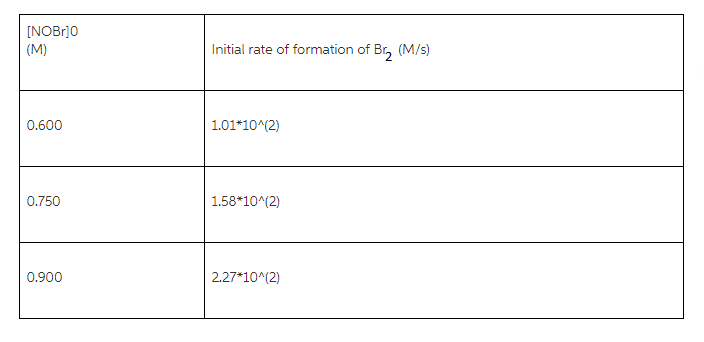# Problem: Consider this initial-rate data at a certain temperature for the reaction described by2 NOBr (g) =&gt; 2 NO (g) + Br2 (g)Determine the value and units of the rate constant k =?

###### FREE Expert Solution
91% (300 ratings)
###### FREE Expert Solution

The rate law can be expressed by:

r = k[reactant]n

From the reaction given:

2 NOBr(g) → 2 NO(g) +Br2(g)

The rate law then becomes:

r = k [NOBr]n

k will be the rate constant. n is the order of the reaction. Before solving for k, we must solve for n.

Substitute the values 1.01x102 for r and 0.600 for reactant in the first rate expression:

91% (300 ratings)###### Problem Details

Consider this initial-rate data at a certain temperature for the reaction described by

2 NOBr (g) => 2 NO (g) + Br(g)

Determine the value and units of the rate constant k =?